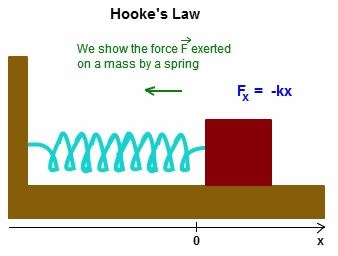# Hookes Law Formula

Hooke’s law states that the force required to extend or compress a spring by some distance is directly proportional to that distance. The stiffness of the spring is a constant factor characteristic. The property of elasticity states that it takes twice the much force to stretch a spring twice as long. This linear dependence of displacement on stretching is known as Hooke’s law. This law is named after 17th-century British physicist Robert Hooke.

It says that the amount of stress we apply on any object is equal to that amount of strain is observed on it, which means Stress Strain.Hooke’s Law Formula is given as

F = -K x

Where,

• F is the amount of force applied in N,
• x is the displacement in the spring in m,
• k is the spring constant or force constant.

Hooke’s law formula can be applied to determine the force constant, displacement, and force in a stretched spring.

### Solved Examples

Example 1

A spring is stretched by 10 cm and has a force constant of 2 cm /dyne. Determine the Force applied.

Solution:

Given parameters are

Force constant k is 2 cm/dyne,

Extension x = 10 cm.

The force applied formula is given by

F = – k x

= – 2 × 10 cm

= – 20 N

Example 2

Determine the force constant if a force of 100 N is stretching a spring by 0.8 m.

Solution:

Given parameters are

Force F = 100 N,

Extension, x = 0.2 m.

The force constant formula is given by

k = – F / x

= – 100 / 0.8

k = – 125 N/m.

For more such valuable equations and formulas stay tuned with BYJU’S!!